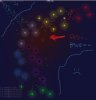# GMLCoordinate Scaling Problem

#### Carbiner

##### Member
Hello!

I'm having a little bit of a coordinate problem.

I'm trying to use a 'heat map' to direct my unit objects. Head for hot sectors, avoid cold ones. It's not really working. They always head for the top left corner. I believe this is a scaling problem, but I don't know what to try next.

The relevant unit code is here:
Code:
``````///scr_unit_heatmap_vector();
// Heat!
var i,ii;
var xx = 0; // Target Coords
var yy = 0;
var heatstuff = 0; // Heat of current cell
var cell_size = controller.cell_size; // How big the cells are
var xcell = x div cell_size; // Our cell
var ycell = y div cell_size;
var side = controller.xcells; // How many cells in each direction
var top = controller.ycells;
var map = controller.heat_map; // The heat map we're using
var cell_checks = 5; // How many cells to check in each direction
var imin = max(0,xcell-cell_checks);
var imax = min(xcell+cell_checks,side);
var iimin = max(0,ycell-cell_checks)
var iimax = min(ycell+cell_checks,top);
var total_heat = ds_grid_get_sum(map,imin,iimin,imax,iimax); // Total heat of the area
if(total_heat == 0) // Making sure we don't get / by 0 errors
{
total_heat = 1;
}
for(i=imin;i<imax;i++)
{
for(ii=iimin;ii<iimax;ii++)
{
heatstuff = ds_grid_get(map,i,ii) / total_heat;
xx += ((i*cell_size)+(cell_size/2))*heatstuff;
yy += ((ii*cell_size)+(cell_size/2))*heatstuff;
}
}
while(xx == x and yy == y) // Make sure our units have a direction
{
xx = x+random(2)-1;
yy = y+random(2)-1;
}
// Find the direction we want to go in
var dir = point_direction(x,y,xx,yy);
var len = 20;
// Set our goto coordinates
inv_x = x+lengthdir_x(len,dir);
inv_y = y+lengthdir_y(len,dir);``````
Here's a picture of what I mean. The units in question are the white ones, and the heat map is drawn over top.I've tried not dividing by the total heat, and by finding the direction off of (0,0) instead of the unit's (x,y), but neither one of those gave a solution, just different, just as bad problems.

If you can help me out, thank you!